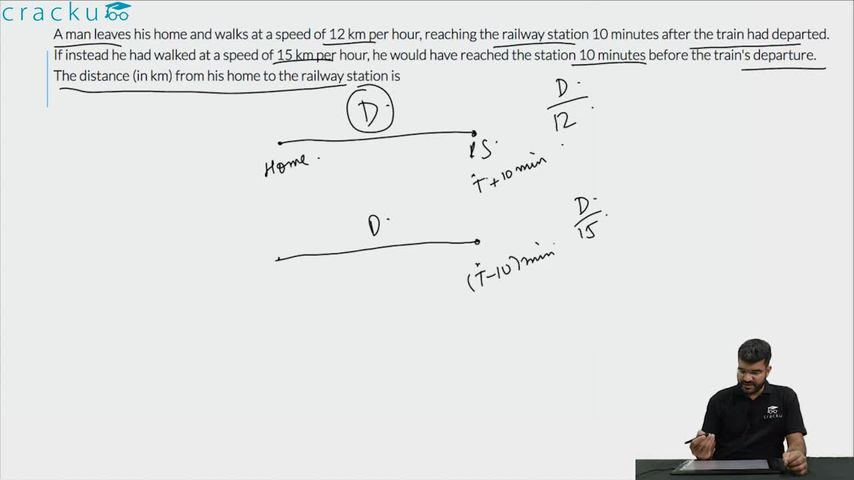Question 70

# A man leaves his home and walks at a speed of 12 km per hour, reaching the railway station 10 minutes after the train had departed. If instead he had walked at a speed of 15 km per hour, he would have reached the station 10 minutes before the train's departure. The distance (in km) from his home to the railway station is

Solution

We see that the man saves 20 minutes by changing his speed from 12 Km/hr to 15 Km/hr.

Let d be the distance

Hence,

$$\frac{d}{12} - \frac{d}{15} = \frac{1}{3}$$

$$\frac{d}{60} = \frac{1}{3}$$

d = 20 Km.

### View Video Solution• All Quant CAT Formulas and shortcuts PDF
• 30+ CAT previous papers with solutions PDF

5 months ago

Thank you sir

1 year ago

thankuu